FreeEMS  0.2.0-SNAPSHOT-282-g9efc524
tableLookup.h File Reference
This graph shows which files directly or indirectly include this file: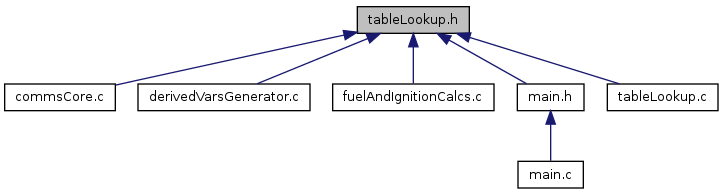Go to the source code of this file.

## Macros

#define EXTERN   extern

## Functions

unsigned short lookupTwoDTableUS (twoDTableUS *, unsigned short) TEXT
unsigned short lookupMainTable (unsigned short, unsigned short, unsigned short) TEXT
unsigned short validateTwoDTable (twoDTableUS *) TEXT
Validate a two D table.
unsigned short validateMainTable (mainTable *) TEXT
Validate a main table.

## Detailed Description

Definition in file tableLookup.h.

## Macro Definition Documentation

 #define EXTERN   extern

Definition at line 48 of file tableLookup.h.

## Function Documentation

 unsigned short lookupTwoDTableUS ( twoDTableUS * Table, unsigned short Value )

Looks up a value from a two D table using interpolation.

Parameters
 Table is a pointer to the table to read from. Value is the position value used to lookup the return value.
Returns
the interpolated value for the position specified

Definition at line 203 of file tableLookup.c.

References twoDTableUS::Axis, and twoDTableUS::Values.

Referenced by calculateFuelAndIgnition(), decodePacketAndRespond(), generateDerivedVars(), and main().

{
/* If never set in the loop, low value will equal high value and will be on the edge of the map */
unsigned short lowAxisValue = Table->Axis;
unsigned short highAxisValue = Table->Axis;
unsigned short lowLookupValue = Table->Values;
unsigned short highLookupValue = Table->Values;
/* Find the bounding axis values and lookup values */
unsigned char Index;
for(Index=0;Index<16;Index++){
if(Table->Axis[Index] < Value){
lowAxisValue = Table->Axis[Index];
lowLookupValue = Table->Values[Index];
}else if(Table->Axis[Index] > Value){
highAxisValue = Table->Axis[Index];
highLookupValue = Table->Values[Index];
break;
}else if(Table->Axis[Index] == Value){
return Table->Values[Index]; // If right on, just return the value
}
}
/* Interpolate and return the value */
return lowLookupValue + (((signed long)((signed long)highLookupValue - lowLookupValue) * (Value - lowAxisValue))/ (highAxisValue - lowAxisValue));
}

Here is the caller graph for this function: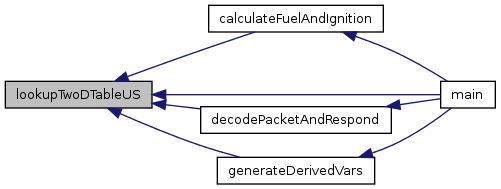unsigned short lookupMainTable ( unsigned short realRPM, unsigned short realLoad, unsigned short locationID )

Looks up a value from a main table using interpolation.

The process :

Take a table with two movable axis sets and two axis lengths, loop to find which pairs of axis values and indexs we are between, interpolate two pairs down to two values, interpolate two values down to one value.

Table size :

To reduce the table size from 19x24 to something smaller, simply reduce the RPMLength and LoadLength fields to lower values. Increasing the size of either axis is not currently possible.

Values outside the table :

Given that the axis lists are in order, a data point outside the table will give the value adjacent to it, and one outside one of the four corners will give the corner value. This is a clean and reasonable behaviour in my opinion.

Reminder : X/RPM is horizontal, Y/Load is vertical

Warning
This function relies on the axis values being a sorted list from low to high. If this is not the case behaviour is undefined and could include memory corruption and engine damage.
Parameters
 realRPM is the current RPM for which a table value is required. realLoad is the current load for which a table value is required. locationID the ID to use to get the memory information to look up the table.

May go back to these: old param RAMPage is the RAM page that the table is stored in. old param Table is a pointer to the table to read from.

Returns
The interpolated value for the location specified.

Definition at line 112 of file tableLookup.c.

Referenced by calculateFuelAndIgnition(), and generateDerivedVars().

{
blockDetails mainTableDetails;
lookupBlockDetails(locationID, &mainTableDetails);
if(!(mainTableDetails.flags & block_is_main_table)){
return 0; // Safe value, always means no fuel or TDC timing.
}
// Leave the rest of the code untouched and keep it more concise
/* Save the RPAGE value for restoration and switch pages. */
unsigned char oldRPage = RPAGE;
RPAGE = mainTableDetails.RAMPage;
/* Find the bounding axis values and indices for RPM */
unsigned char lowRPMIndex = 0;
unsigned char highRPMIndex = Table->RPMLength - 1;
/* If never set in the loop, low value will equal high value and will be on the edge of the map */
unsigned short lowRPMValue = Table->RPM;
unsigned short highRPMValue = Table->RPM[Table->RPMLength -1];
unsigned char RPMIndex;
for(RPMIndex=0;RPMIndex<Table->RPMLength;RPMIndex++){
if(Table->RPM[RPMIndex] < realRPM){
lowRPMValue = Table->RPM[RPMIndex];
lowRPMIndex = RPMIndex;
}else if(Table->RPM[RPMIndex] > realRPM){
highRPMValue = Table->RPM[RPMIndex];
highRPMIndex = RPMIndex;
break;
}else if(Table->RPM[RPMIndex] == realRPM){
lowRPMValue = Table->RPM[RPMIndex];
highRPMValue = Table->RPM[RPMIndex];
lowRPMIndex = RPMIndex;
highRPMIndex = RPMIndex;
break;
}
}
/* Find the bounding cell values and indices for Load */
/* If never set in the loop, low value will equal high value and will be on the edge of the map */
break;
break;
}
}
/* Obtain the four corners surrounding the spot of interest */
/* Restore the RAM page before doing the math */
RPAGE = oldRPage;
/* Find the two side values to interpolate between by interpolation */
/* Interpolate between the two side values and return the result */
}

Here is the call graph for this function: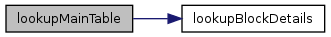Here is the caller graph for this function: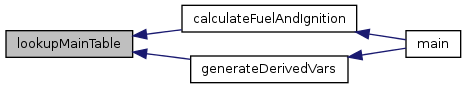unsigned short validateTwoDTable ( twoDTableUS * Table )

Validate a two D table.

Check that the order of the axis values is correct and therefore that the table is valid too.

Parameters
 Table is a pointer to the table to be validated.
Returns
An error code. Zero means success, anything else is a failure.

Definition at line 280 of file tableLookup.c.

References twoDTableUS::Axis, invalidTwoDTableAxisOrder, and TWODTABLEUS_LENGTH.

Referenced by decodePacketAndRespond().

{
/* Check the order of the axis */
unsigned char i;
for(i=0;i<(TWODTABLEUS_LENGTH - 1);i++){
if(Table->Axis[i] >= Table->Axis[i+1]){
}
}
return 0;
}

Here is the caller graph for this function: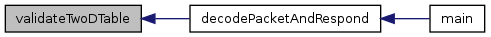unsigned short validateMainTable ( mainTable * Table )

Validate a main table.

Check that the configuration of the table is valid. Assumes pages are correctly set.

Todo:
more detail here....
Parameters
 Table is a pointer to the table to be validated.
Returns
An error code. Zero means success, anything else is a failure.

Definition at line 240 of file tableLookup.c.

Referenced by decodePacketAndRespond().

{
/* If required and only if required extend this to take r and f pages and check */
/* any main table, not just a freshly received untrusted ones in linear space */
}else if((Table->RPMLength * Table->LoadLength) > MAINTABLE_MAX_MAIN_LENGTH){
}else{
/* Check the order of the RPM axis */
unsigned char i;
for(i=0;i<(Table->RPMLength - 1);i++){
if(Table->RPM[i] >= Table->RPM[i+1]){
}
}
/* Check the order of the Load axis */
unsigned char j;